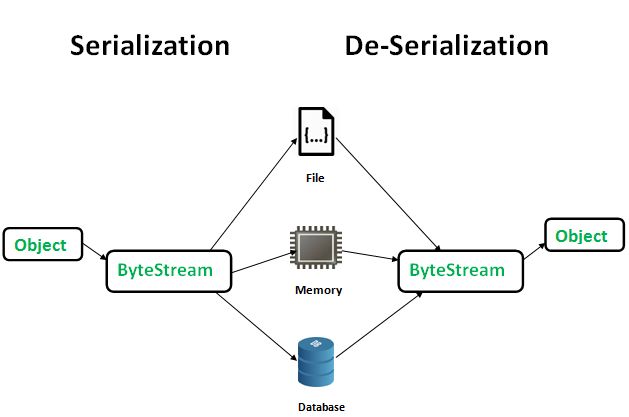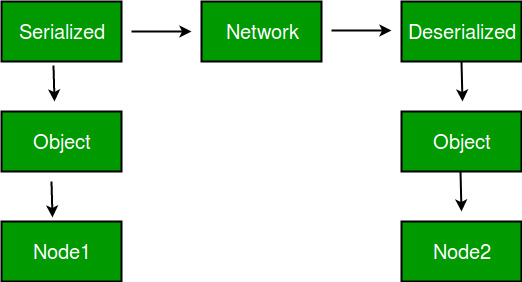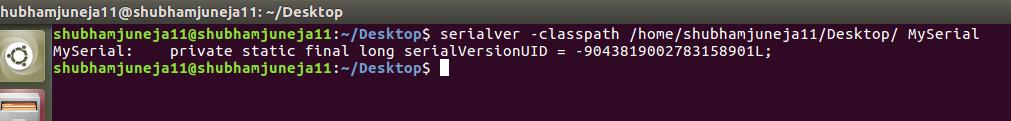GeeksforGeeks App
Open AppBrowser
Continue

# Serialization and Deserialization in Java with Example

Serialization is a mechanism of converting the state of an object into a byte stream. Deserialization is the reverse process where the byte stream is used to recreate the actual Java object in memory. This mechanism is used to persist the object.The byte stream created is platform independent. So, the object serialized on one platform can be deserialized on a different platform. To make a Java object serializable we implement the java.io.Serializable interface. The ObjectOutputStream class contains writeObject() method for serializing an Object.

```public final void writeObject(Object obj)
throws IOException```

The ObjectInputStream class contains readObject() method for deserializing an object.

```public final Object readObject()
throws IOException,
ClassNotFoundException```

1. To save/persist state of an object.
2. To travel an object across a network.Only the objects of those classes can be serialized which are implementing java.io.Serializable interface. Serializable is a marker interface (has no data member and method). It is used to “mark” java classes so that objects of these classes may get certain capability. Other examples of marker interfaces are:- Cloneable and Remote.

Points to remember

1. If a parent class has implemented Serializable interface then child class doesn’t need to implement it but vice-versa is not true. 2. Only non-static data members are saved via Serialization process.
3. Static data members and transient data members are not saved via Serialization process. So, if you don’t want to save value of a non-static data member then make it transient.
4. Constructor of object is never called when an object is deserialized.
5. Associated objects must be implementing Serializable interface. Example :

```class A implements Serializable{

// B also implements Serializable
// interface.
B ob=new B();
}```

SerialVersionUID The Serialization runtime associates a version number with each Serializable class called a SerialVersionUID, which is used during Deserialization to verify that sender and receiver of a serialized object have loaded classes for that object which are compatible with respect to serialization. If the receiver has loaded a class for the object that has different UID than that of corresponding sender’s class, the Deserialization will result in an InvalidClassException

A Serializable class can declare its own UID explicitly by declaring a field name. It must be static, final and of type long. i.e- ANY-ACCESS-MODIFIER static final long serialVersionUID=42L; If a serializable class doesn’t explicitly declare a serialVersionUID, then the serialization runtime will calculate a default one for that class based on various aspects of class, as described in Java Object Serialization Specification. However it is strongly recommended that all serializable classes explicitly declare serialVersionUID value, since its computation is highly sensitive to class details that may vary depending on compiler implementations, any change in class or using different id may affect the serialized data. It is also recommended to use private modifier for UID since it is not useful as inherited member. serialver The serialver is a tool that comes with JDK. It is used to get serialVersionUID number for Java classes.

You can run the following command to get serialVersionUID serialver [-classpath classpath] [-show] [classname…]Example 1:

## Java

 `// Java code for serialization and deserialization``// of a Java object``import` `java.io.*;` `class` `Demo ``implements` `java.io.Serializable``{``    ``public` `int` `a;``    ``public` `String b;` `    ``// Default constructor``    ``public` `Demo(``int` `a, String b)``    ``{``        ``this``.a = a;``        ``this``.b = b;``    ``}` `}` `class` `Test``{``    ``public` `static` `void` `main(String[] args)``    ``{  ``        ``Demo object = ``new` `Demo(``1``, "geeksforgeeks");``        ``String filename = "file.ser";``        ` `        ``// Serialization``        ``try``        ``{  ``            ``//Saving of object in a file``            ``FileOutputStream file = ``new` `FileOutputStream(filename);``            ``ObjectOutputStream out = ``new` `ObjectOutputStream(file);``            ` `            ``// Method for serialization of object``            ``out.writeObject(object);``            ` `            ``out.close();``            ``file.close();``            ` `            ``System.out.println("Object has been serialized");` `        ``}``        ` `        ``catch``(IOException ex)``        ``{``            ``System.out.println("IOException is caught");``        ``}`  `        ``Demo object1 = ``null``;` `        ``// Deserialization``        ``try``        ``{  ``            ``// Reading the object from a file``            ``FileInputStream file = ``new` `FileInputStream(filename);``            ``ObjectInputStream in = ``new` `ObjectInputStream(file);``            ` `            ``// Method for deserialization of object``            ``object1 = (Demo)in.readObject();``            ` `            ``in.close();``            ``file.close();``            ` `            ``System.out.println("Object has been deserialized ");``            ``System.out.println("a = " + object1.a);``            ``System.out.println("b = " + object1.b);``        ``}``        ` `        ``catch``(IOException ex)``        ``{``            ``System.out.println("IOException is caught");``        ``}``        ` `        ``catch``(ClassNotFoundException ex)``        ``{``            ``System.out.println("ClassNotFoundException is caught");``        ``}` `    ``}``}`

Output :

```Object has been serialized
Object has been deserialized
a = 1
b = geeksforgeeks```

Example 2:

## Java

 `// Java code for serialization and deserialization``// of a Java object``import` `java.io.*;` `class` `Emp ``implements` `Serializable {``private` `static` `final` `long` `serialversionUID =``                                 ``129348938L;``    ``transient` `int` `a;``    ``static` `int` `b;``    ``String name;``    ``int` `age;` `    ``// Default constructor``public` `Emp(String name, ``int` `age, ``int` `a, ``int` `b)``    ``{``        ``this``.name = name;``        ``this``.age = age;``        ``this``.a = a;``        ``this``.b = b;``    ``}` `}` `public` `class` `SerialExample {``public` `static` `void` `printdata(Emp object1)``    ``{` `        ``System.out.println("name = " + object1.name);``        ``System.out.println("age = " + object1.age);``        ``System.out.println("a = " + object1.a);``        ``System.out.println("b = " + object1.b);``    ``}` `public` `static` `void` `main(String[] args)``    ``{``        ``Emp object = ``new` `Emp("ab", ``20``, ``2``, ``1000``);``        ``String filename = "shubham.txt";` `        ``// Serialization``        ``try` `{` `            ``// Saving of object in a file``            ``FileOutputStream file = ``new` `FileOutputStream``                                           ``(filename);``            ``ObjectOutputStream out = ``new` `ObjectOutputStream``                                           ``(file);` `            ``// Method for serialization of object``            ``out.writeObject(object);` `            ``out.close();``            ``file.close();` `            ``System.out.println("Object has been serialized\n"``                              ``+ "Data before Deserialization.");``            ``printdata(object);` `            ``// value of static variable changed``            ``object.b = ``2000``;``        ``}` `        ``catch` `(IOException ex) {``            ``System.out.println("IOException is caught");``        ``}` `        ``object = ``null``;` `        ``// Deserialization``        ``try` `{` `            ``// Reading the object from a file``            ``FileInputStream file = ``new` `FileInputStream``                                         ``(filename);``            ``ObjectInputStream in = ``new` `ObjectInputStream``                                         ``(file);` `            ``// Method for deserialization of object``            ``object = (Emp)in.readObject();` `            ``in.close();``            ``file.close();``            ``System.out.println("Object has been deserialized\n"``                                ``+ "Data after Deserialization.");``            ``printdata(object);` `            ``// System.out.println("z = " + object1.z);``        ``}` `        ``catch` `(IOException ex) {``            ``System.out.println("IOException is caught");``        ``}` `        ``catch` `(ClassNotFoundException ex) {``            ``System.out.println("ClassNotFoundException" +``                                ``" is caught");``        ``}``    ``}``}`

Output:

```Object has been serialized
Data before Deserialization.
name = ab
age = 20
a = 2
b = 1000
Object has been deserialized
Data after Deserialization.
name = ab
age = 20
a = 0
b = 2000```

Description for Output: You have seen while deserializing the object the values of a and b has changed. The reason being a was marked as transient and b was static.

In case of transient variables:- A variable defined with transient keyword is not serialized during serialization process.This variable will be initialized with default value during deserialization. (e.g: for objects it is null, for int it is 0).

In case of static Variables:- A variable defined with static keyword is not serialized during serialization process.This variable will be loaded with current value defined in the class during deserialization.

Transient Vs Final:
final variables will be participated into serialization directly by their values.
Hence declaring a final variable as transient there is no use.
//the compiler assign the value to final variable

example:

```final int x= 10;
int y = 20;
System.out.println(x);// compiler will replace this as System.out.println(10)->10
because x is final.
System.out.println(y);//20
```

Example 3:

## Java

 `//java code for final with transient` `import` `java.io.*;`  `class` `Dog ``implements` `Serializable{``          ``int` `i=``10``;``          ``transient` `final` `int` `j=``20``;``}``class` `GFG {``    ``public` `static` `void` `main (String[] args)``throws` `IOException,ClassNotFoundException``    ``{``          ``Dog d1=``new` `Dog();``          ``//Serialization started``          ``System.out.println(``"serialization started"``);``          ``FileOutputStream fos= ``new` `FileOutputStream(``"abc.ser"``);``          ``ObjectOutputStream oos=``new` `ObjectOutputStream(fos);``          ``oos.writeObject(d1);``          ``System.out.println(``"Serialization ended"``);``      ` `          ``//Deserialization started``          ``System.out.println(``"Deserialization started"``);``          ``FileInputStream fis=``new` `FileInputStream(``"abc.ser"``);``          ``ObjectInputStream ois=``new` `ObjectInputStream(fis);``          ``Dog d2=(Dog) ois.readObject();``          ``System.out.println(``"Deserialization ended"``);``          ``System.out.println(``"Dog object data"``);``          ``//final result``          ``System.out.println(d2.i+``"\t"` `+d2.j);``    ``}``}`

Output

```serialization started
Serialization ended
Deserialization started
Deserialization ended
Dog object data
10    20```

My Personal Notes arrow_drop_up
Related Tutorials